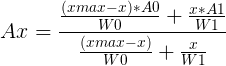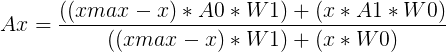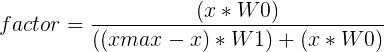The DS GPU interpolation May 15th 2019, by Arisotura I said a while back that I'd be writing about how the DS GPU interpolates vertex attributes when drawing polygons, so, here we go. As explained in our previous technical post, vertex attributes are interpolated twice: once along the polygon edges, and once along the scanline being drawn. The details of how those are interpolated is where the meat is. A typical 3D rasterizer will perform perspective-correct interpolation, which is meant to take perspective distortion into account when drawing a polygon that has been projected to 2D screen space. There are early rasterizers that use different interpolation types, for example, the Playstation does affine texture mapping. In the case of the DS, it does perspective-correct texture mapping and shading. The basics This is the canonical formula for perspective-correct interpolation over a given span:where: - x is the position within the span, units don't matter (as long as they're consistent with xmax) - xmax is the length of the span - A0 and A1 are the attributes at each end of the span - W0 and W1 are the associated W coordinates - Ax is the interpolated attribute at position x Rasterizers implement this formula in one way or another. For example, it is typical for software rasterizers to start by dividing all vertex attributes by W, so those can be interpolated linearly, then divide them by the W reciprocal to recover the correct values for each pixel. I don't know much about how typical GPUs implement this. However, all the research I did back then for melonDS's renderer gave me a lot of insight over this. Regarding x and xmax, the DS GPU keeps things simple. When interpolating vertically (ie across polygon edges), those are either Y or X positions, depending on the slope of the edge. For X-major edges, X positions are a lot more precise than Y positions, so this makes sense. When interpolating horizontally (ie over a scanline span), those are X positions. As said above, units for x and xmax don't matter, so the pixel coordinates are used as-is. The interpolation calculation itself is where this gets interesting. I eventually figured out how it works after observing interpolation of W values over large spans: those always changed by increments of 1/256th of the difference between the W values. For example, given W values 0x1000 to 0x2000 over a span of 256 pixels, the canonical formula had it begin like: 0x1000, 0x1008, 0x1010, 0x1018, 0x1020... On the DS, it was 0x1000, 0x1000, 0x1010, 0x1010, 0x1020... This was also the case with other ranges, like 0x1001-0x2001, 0x1007-0x2007, etc, further straying away from the canonical formula. This had me scratching my head for a while until I finally figured it out: the DS GPU precalculates an interpolation factor with limited precision, then uses that to interpolate vertex attributes linearly. So, how does that work? Well, the canonical formula can be transposed to this simpler, less division-y version:The DS simply sets A0 to zero and A1 to 1. The precision is 8 bits of fractional part along scanline spans (hence the effect observed above), and 9 bits along polygon edges. Thus, we have:With this factor, we can interpolate vertex attributes linearly quite quickly, resulting in a pretty good (but imperfect) approximation of perspective-correct interpolation. There are some interesting quirks about all this, though. Divider details The DS uses an unsigned 32-bit divider to perform the division, which induces some extra quirks. Those quirks become evident when using tiny W values. For example, if you draw a polygon with W values going from 0x1000 to 0xF000 on the left side, and 0x0001 to 0x000F on the right side, you will get distortion on the right side. In the formula above: - W0 and W1 are 16-bit (those are 'normalized' during polygon setup: they're shifted left or right by 4 until they all fit the 16-bit range as much as possible) - x fits within 8 bits - (xmax-x) may take up 9 bits, if viewport transform has overflowed The denominator takes up, at most, 26 bits. This is okay. The numerator takes 24 bits. Along scanline spans, we add 8 bits of fractional part, bringing it to 32 bits. However, along polygon edges, we add 9 bits, which brings it to 33 bits. Oops. For this reason, when interpolating along polygon edges, there's some weird adjustment made to W values, so those fit in 15 bits. This is best described by the following code: if ((w0 & 0x1) && !(w1 & 0x1)) {    w0_numerator = w0 - 1;    w0_denominator = w0 + 1;    w1_denominator = w1; } else {    w0_numerator = w0 & 0xFFFE;    w0_denominator = w0 & 0xFFFE;    w1_denominator = w1 & 0xFFFE; } This is namely responsible for the dents in SM64DS's level select buttons. Alternate linear-interpolation path Due to the limited fixed-point precision, the method described above isn't suitable for 2D polygons, those could end up with misplaced textures. This is where the DS has another trick in store: for those cases, it directly does linear interpolation, completely bypassing the perspective correction math. Technically, it checks the W values at the ends of the span. If those are equal, and have low-order bits cleared (bit0-6 along scanline spans, bit1-6 along polygon edges), the alternate linear-interpolation path is used. I'm not sure why low-order bits have to be cleared, maybe that is to guard against precision errors? Interpolator note The interpolators in the DS GPU, similarly to the divider, only work with unsigned numbers. The basic operation would be: val = a + (((b-a) * x) / xmax); What if a is greater than b? (b-a) would end up negative. To avoid this, it does the operation in reverse: if (a < b) val = a + (((b-a) * x) / xmax); else if (a > b) val = b + (((a-b) * (xmax-x)) / xmax); Side note We're coding epic shit for melonDS 0.8. Not telling much more though, that'll be a surprise :) 7 comments have been posted.
 branchus says: May 16th 2019 don't know much about the content, but good work, waiting for the 0.8
 QuickProcess says: May 16th 2019 Thank you for this. From USA
 cyber says: May 16th 2019 Thank you for making an amazing DS emulator. You are doing amazing work! Looking forward to 0.8
 Zinx says: May 16th 2019 I hope 0.8 gonna come soon pretty good stuff.